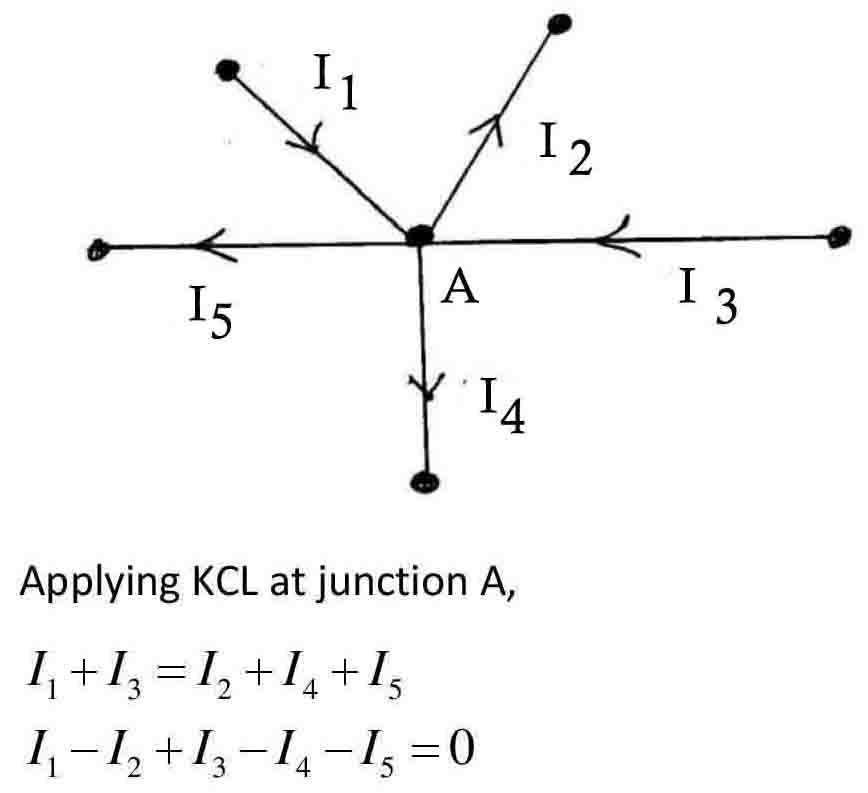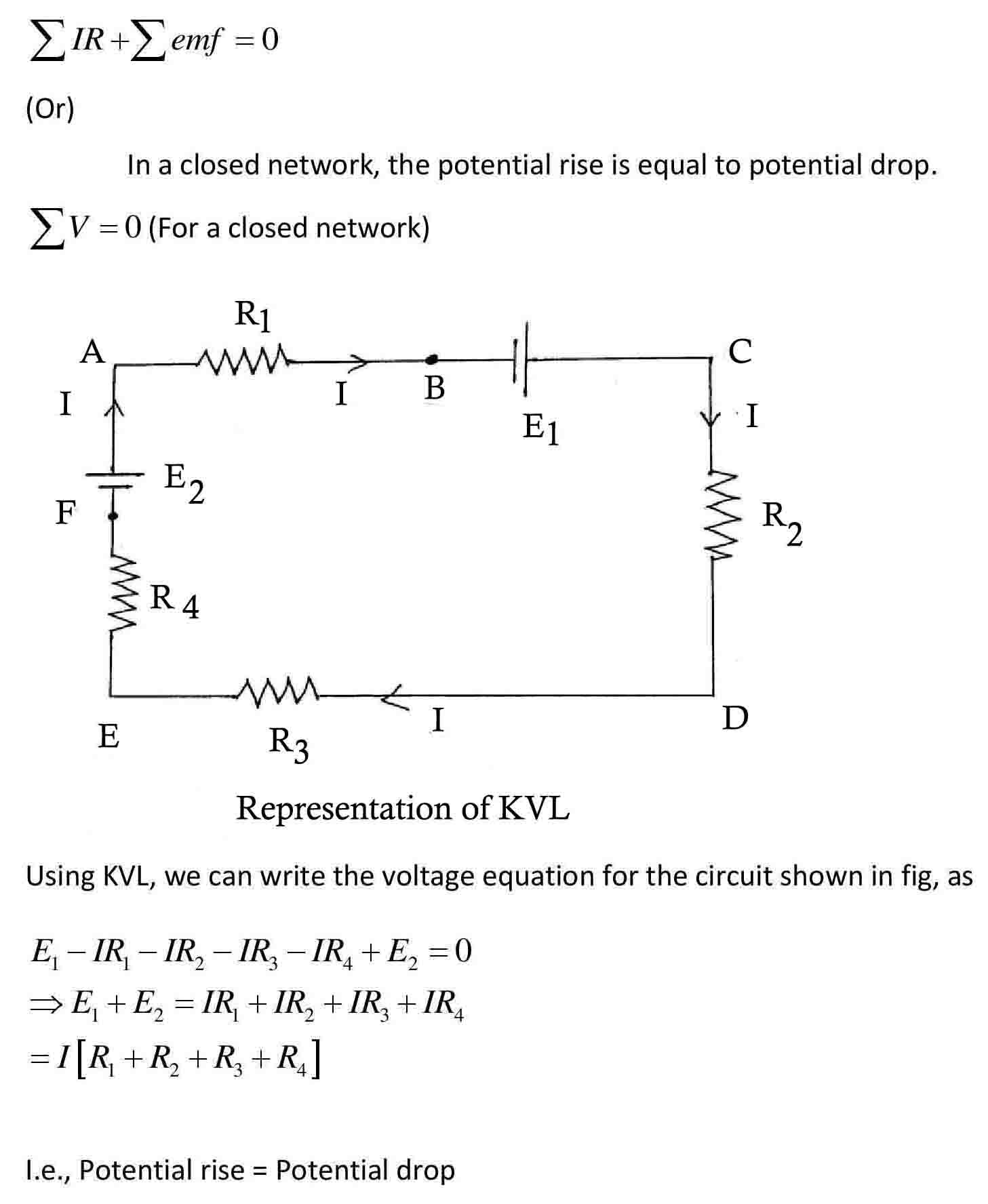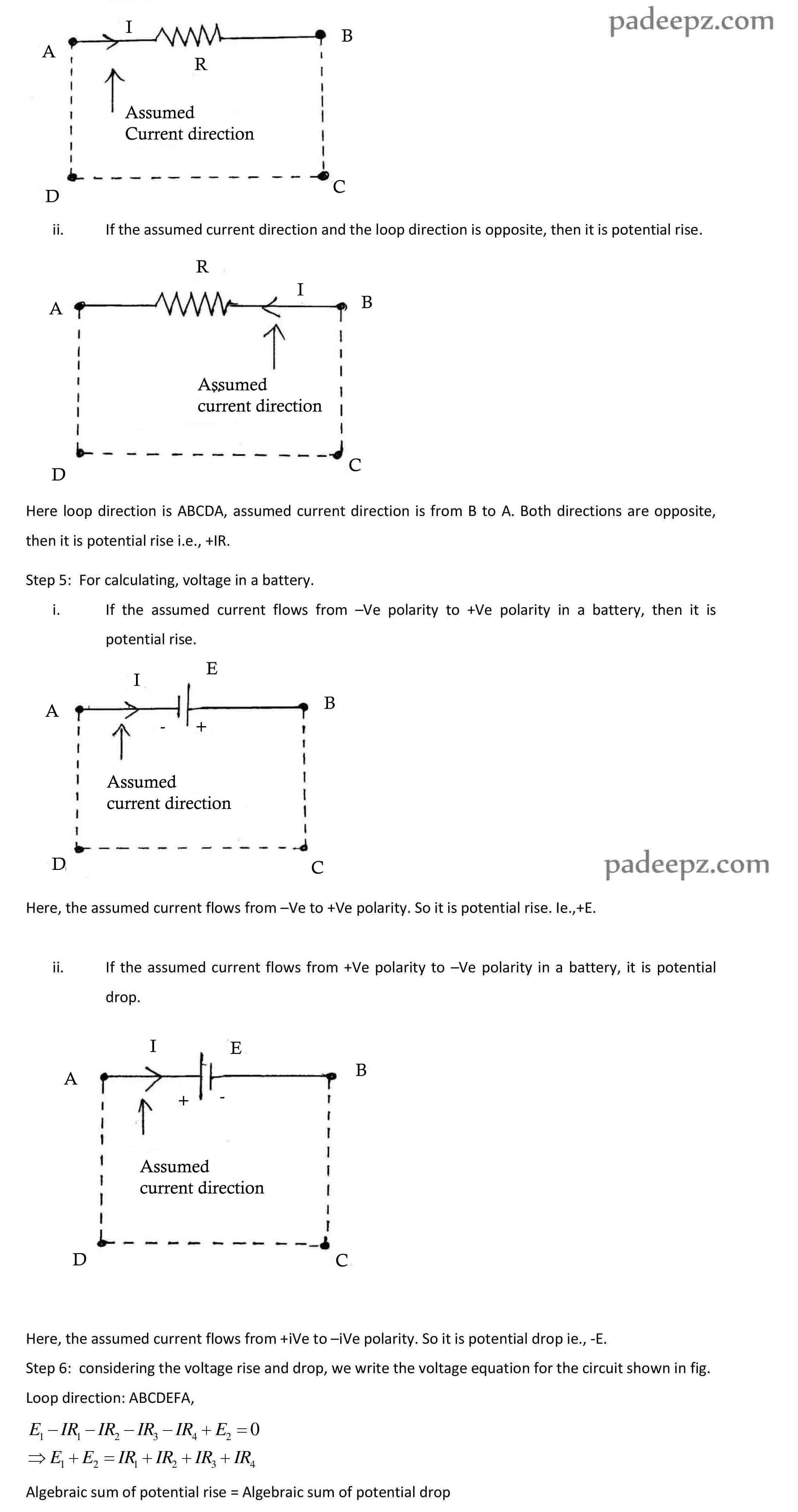# Padeepz E-Learning Materials EE6201 Circuit Theory

Padeepz E-Learning Materials EE6201 Circuit Theory we have provided the sample materials in this page. If you like the sample and want to buy the full subject the procedure is also provided in this page.

## Kirchoff’s Laws

Kirchoff’s Current Laws

Statement

At a junction, total current flowing towards the junction is equal to total current flowing away from the junction.

(or)

The algebraic sum of current meeting at a junction or node is zero.Kirchoff’s Voltage Laws

Statement

In any closed network, the algebraic sum of product of current and resistance (Voltage drop) across the circuit elements of any closed path is equal to the algebraic sum of the emf’s in the path.Formation of KVL Equation

Step 1:  Give names for each and every possible nodes.

Step 2:  Write the loop direction in terms of node (ABCDA)

Step 3:  Assume the current direction for each branch.

Step 4:  For calculating the voltage across a resistor.

If the assumed current direction and the loop direction is same, then it is potential drop. Loop direction is ABCDA, assumed current direction is from A to B. Both directions are same, then it is potential drop i.e., -IR.Note:    For ‘n’ number of closed loops (Meshes), there will be ‘n’ number of loops (mesh) currents and hence we need ‘n’ number of equations.